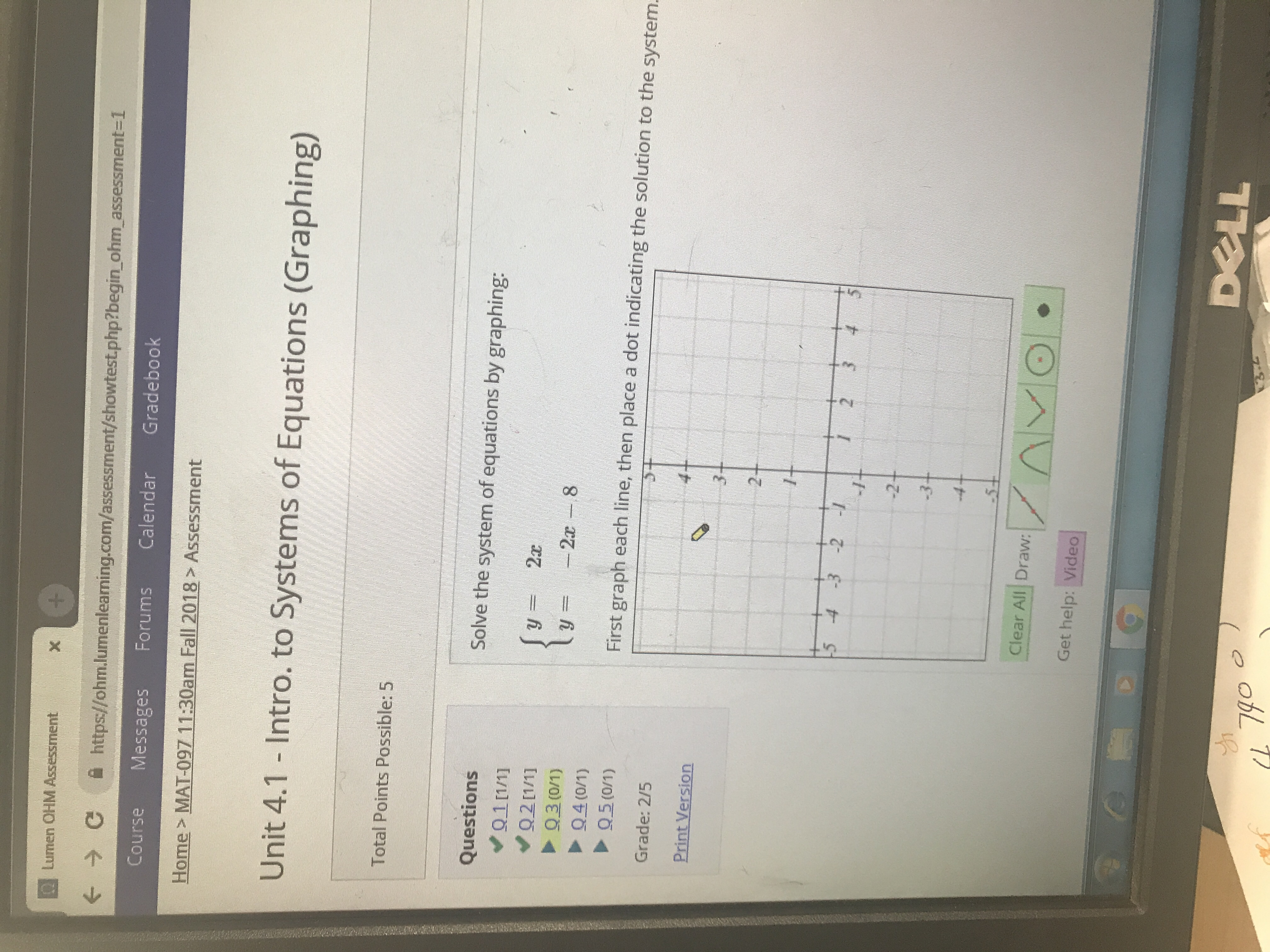# X困Lumen OHM AssessmentСhttps://ohm.lumenleaming.com/assessment/showtest.php?begin ohm, assessment-1Course Messages Forums Calendar GradebookHome> MAT-097 11:30am Fall 2018 AssessmentUnit 4.1 - Intro. to Systems of Equations (Graphing)Total Points Possible: 5QuestionsSolve the system of equations by graphing:94 (0/1)First graph each line, then place a dot indicating the solution to the systemGrade: 2/5Print Version-4Clear All Draw:Get help: Video

Questionhelp_outlineImage TranscriptioncloseX 困Lumen OHM Assessment С https://ohm.lumenleaming.com/assessment/showtest.php?begin ohm, assessment-1 Course Messages Forums Calendar Gradebook Home> MAT-097 11:30am Fall 2018 Assessment Unit 4.1 - Intro. to Systems of Equations (Graphing) Total Points Possible: 5 Questions Solve the system of equations by graphing: 94 (0/1) First graph each line, then place a dot indicating the solution to the system Grade: 2/5 Print Version -4 Clear All Draw: Get help: Video fullscreen
check_circle

Step 1

Given:

The system of equation is y=2x and y=-2x-8.

Step 2

Make the table for contructing the graph:

For x=1, y=2*1=2

For x=2, y=2*2=4

For x=-1, y=2*-1=-2

For x=-2,y=2*-2=-4

So, the points are (-2,-4),(-1,-2),(1,2) and (2,4)

Step 3

Make the table for constructing the graph:

For x=-2, y=-2(-2)-8=-4

...

### Want to see the full answer?

See Solution

#### Want to see this answer and more?

Solutions are written by subject experts who are available 24/7. Questions are typically answered within 1 hour.*

See Solution
*Response times may vary by subject and question.
Tagged in

### Algebra